# Al2O3对煤粉中CaO固硫的热力学影响研究Thermodynamic Effect of Al2O3 on Sulfur Fixation by CaO in Pulverized Coal Combustion

• 全文下载: PDF(2010KB)    PP.198-202   DOI: 10.12677/HJCET.2019.93029
• 下载量: 195  浏览量: 291   科研立项经费支持

Based on the experimental study on the sulfur fixation of calcium-based sulfur fixation agent CaO on pulverized coal and the thermodynamic calculation on the sulfur fixation system, it was found that the sulfur fixation product CaSO4 was unstable at high temperature. However, compound products would be formed with the addition of Al2O3, and it could inhibit the decomposition of CaSO4, thus increase the sulfur fixation efficiency of CaO on pulverized coal at high temperature.

1. 引言

2. 无Al2O3存在时的CaSO4热稳定性的实验研究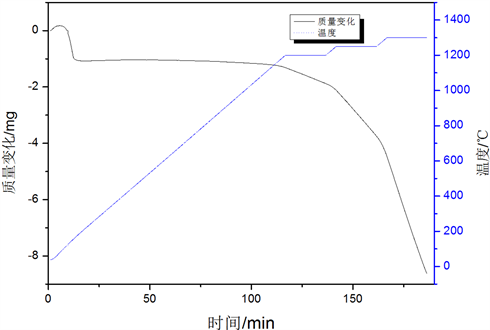Figure 1. TG curve of CaSO4 decomposition

3. Al2O3-CaO-CaSO4体系的热力学计算

3.1. Factsage软件计算过程概述

3.2. 计算结果

3.3. 计算结果分析

$\text{CaO}+{\text{Al}}_{\text{2}}{\text{O}}_{\text{3}}\to \text{CaO}\cdot {\text{Al}}_{\text{2}}{\text{O}}_{\text{3}}$ (1)

$\text{CaO}+{\text{6Al}}_{\text{2}}{\text{O}}_{\text{3}}\to \text{CaO}\cdot {\text{6Al}}_{\text{2}}{\text{O}}_{\text{3}}$ (2)

$\text{CaO}+{\text{2Al}}_{\text{2}}{\text{O}}_{\text{3}}\to \text{CaO}\cdot {\text{2Al}}_{\text{2}}{\text{O}}_{\text{3}}$ (3)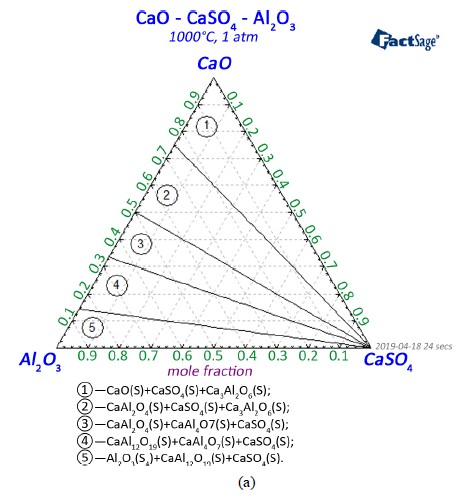(a)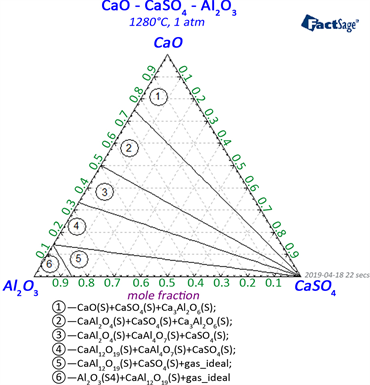(b)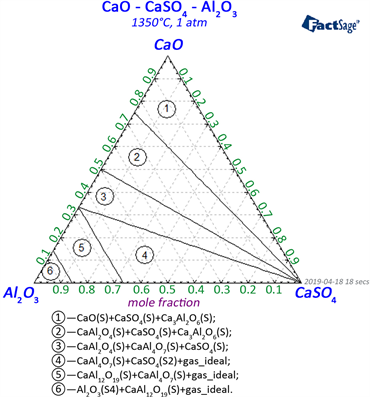(c)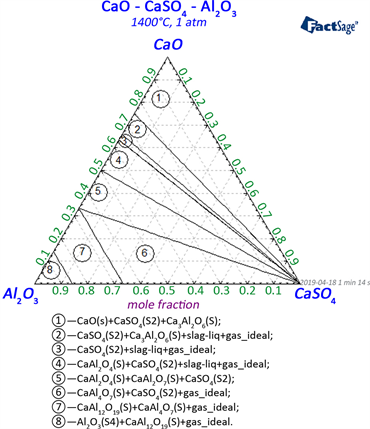(d)

Figure 2. Calculation results of CaO-CaSO4-Al2O3 system at different temperature

$\text{3CaO}+{\text{Al}}_{\text{2}}{\text{O}}_{\text{3}}\to \text{3CaO}\cdot {\text{Al}}_{\text{2}}{\text{O}}_{\text{3}}$ (4)

${\text{CaSO}}_{\text{4}}\to \text{CaO}+{\text{SO}}_{\text{2}}+\text{1}/{\text{2O}}_{\text{2}}$ (5)

4. 结论

1) 单一存在的CaSO4在1200℃时开始分解，到1400℃时大量分解。

2) Al2O3-CaO-CaSO4复合体系中在1280℃时CaSO4才开始分解，温度升高到1400℃后由于体系出现液相并包裹或覆盖在CaSO4表面，从而抑制了其分解，使固硫产物在Al2O3存在时能够稳定存在，保证了CaO的高温固硫效果。

  张生芹, 谢兵. MnO2对煤粉中CaO固硫效率影响机理[J]. 环境工程学报, 2012, 6(1): 4-7.  杨剑锋. 钙基复合添加剂的固硫机理及其对固硫产物的影响[D]: [硕士学位论文]. 武汉: 华中科技大学, 2004.  Daniel, C.B. and Amir, A. (1981) Sulfur Pollution from Coal Combustion. Effect of the Mineral Components of Coal on the Thermal Stabitities of Sulfated Ash and Calcium Sulfate. Environment Science & Technology, 15, 288-298. https://doi.org/10.1021/es00085a003  武增华, 姚绍军, 郭锋, 等. 氧化物添加剂对CaSO4高温稳定性的影响[J]. 煤炭转化, 2002, 25(22): 71-73.  杨晓东, 武建军, 薛可轶, 等. 添加剂抑制CaSO4高温分解的TG-FTIR研究(I)氧化物系列[J].. 煤炭转化, 2003, 26(4): 69-72.